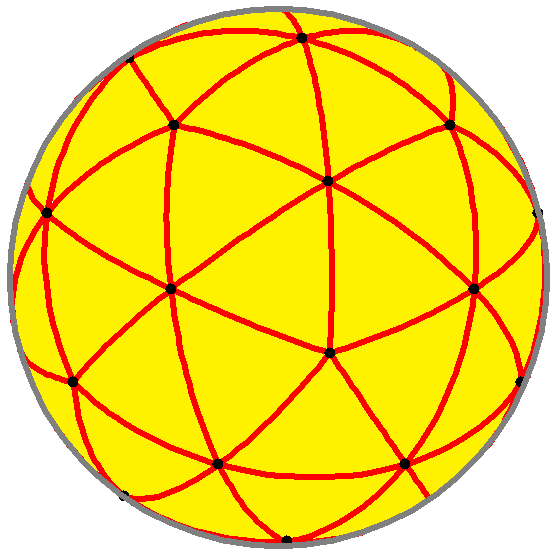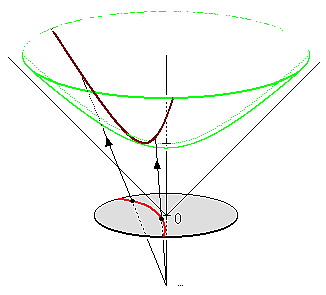# Folding Animated Hyperbolic & Spherical Tilings

In a previous post, I talked about generating interesting pictures by iterated folding operations. In this post I’m going to use another type of fold to draw both hyperbolic and spherical tilings. If you are already familiar with hyperbolic geometry and tilings, skip the next few paragraphs! There’s code down at the bottom.

In order to simplify what we’re talking about, we can limit ourselves to tilings of right-angled triangles. In the Euclidean plane, there are two ways to tile the plane this way. One can be identified with a regular tiling of squares, and the other with a regular tiling of hexagons (or equilateral triangles).A dodecahedral tiling

There are no other ways to tile the plane like this. But instead of tiling the Euclidean plane, we can look toward other geometries. One you are probably familiar with is spherical geometry: instead of drawing lines on a flat plane, we draw arcs on a sphere. Inside the spherical geometry, portions of great circles are actually straight lines. While things look a bit different, you can still build right triangles, and tile the sphere with them. On the sphere, there are few more tilings!

In a sense, the spherical geometry is bending every straight line a bit towards every other straight line. If you extend a line segment, it will intersect every other line, “around the back” of the sphere. You can convince yourself of this with some patient time with a globe.Two views of a hyperboloid

There’s another geometry that goes in the “other direction”: it bends every straight line away from every other. This is the mysterious hyperbolic geometry. It’s called hyperbolic by analogy to the spherical geometry: you can draw lines as parts of hyperbolas, on one sheet of a hyperboloid.

In this geometry, there are even more tilings (an infinite number, in fact). You can fit as many identical right triangles around a vertex as you like, and the resulting polygon can tile the plane. There is, in a sense, “more space” to move around in. If you draw two straight lines, they may intersect- but they might not intersect at all, no matter how far you extend them. There’s ‘more’ space for the lines to go, and they tend to bend away from each other.

While these geometries both “live” in three-dimensional objects (spheres and hyperboloids) they can be modeled in the plane. In the case of spherical geometry, you can simply stereographically project the sphere onto the plane. Straight lines (arcs) on the sphere become circles in the plane. Further away from the origin, objects look bigger (even if they’re the same size on the sphere).Poincaré projection

The model we are using of hyperbolic geometry is called the Poincaré Disk. It gathers the entirety of the hyperbolic space into a circle in the plane. It is formed similarly to the stereographic projection: it projects points on the hyperboloid down onto the plane by casting a ray from a point underneath.Three hyperbolic lines

Straight lines (hyperbolas) in the hyperbolic geometry are drawn as circles in the plane. Further away from the origin (and closer to the edge of the disk), objects look smaller (even though they’re the same size on the hyperboloid). Angles are preserved, so (for instance) two lines that appear to be perpendicular when measured by Euclidean standards actually are perpendicular.

Okay. Long-winded math over, let’s start folding things. Like the previous post, we’re going to be mirroring pixels across a boundary. In this case, we are going to keep track of the number of times each point is actually moved by a folding operation. If a point is folded, we increment a variable; once we’re done, we use the value to color the pixel.

To begin, we do two folds across the X and Y axes. You can adjust the orientation of the fold axes to see how it behaves. The upper-right quadrant stays black because points there are never moved. Points in the lower left are folded twice, and so are also black. The other two are folded exactly once, so they turn gray.

In the Poincaré disk model of hyperbolic geometry, a straight line is drawn as a portion of a generalized circle (generalized, because the center of the circle can be “at infinity”, and it looks like a line through the origin). To reflect across a hyperbolic line you actually invert across the circle. Inside the hyperbolic geometry, distances aren’t affected by inversion, even if they look like they are in our model. So, using the circle inversion idea, we define a fold “across” a circle. If a point is outside the circle, it is unaffected, but if it is inside, it is “folded” to the outside with a circle inversion.

Here, we are folding across two lines and a circle. From the point of view of the Poincaré model, all three folds are across straight lines (or, alternatively, there are three circle inversions, and two of the circles have a center at infinity). You could do this with three suitably chosen circles, too.

Now if we just apply the folds a few more times, a new picture starts to emerge: it’s a Poincaré disk! These three folds are actually generating a hyperbolic geometry. Adjust the number of iterations to see how the circle inversions successively copy and smoosh the shapes into a steady progression towards the perimeter. These shapes are all right triangles, and they are all the same shape and size.

In the Poincaré disk model, not every arc is a straight line: it has to be perpendicular to the edge of the disk. So you’ll notice that as you move the inverting circle, the generated disk shifts and changes shape. With some more math, you can work back from the disk to find circles that will generate it. Many choices of circle don’t result in a uniform tiling at all. More math can tame this as well, and generate tilings based on how many tiles should fit around each vertex.

You might have noticed that the picture changes quite a lot if the inverting circle actually includes the origin. The tiling suddenly doesn’t approach a limit: it fills the entire plane with a finite number of tiles. The geometric reason is simply that, if you start inside a circle, the inversion will make the tile bigger and bigger, rather than smaller and smaller (like it did when the origin was outside the circle). The result here is actually a model of spherical geometry, and we’ve generated a tiling of the sphere. Conveniently, reflections in spherical geometry are also modeled by circle inversions in the plane.

When the origin is exactly on the circumference of the circle, we get a third case. There are an infinite number of tiles, but unlike the hyperbolic case, they march towards the origin. This looks pretty unfamiliar. It’s definitely not spherical, and it doesn’t look much like the hyperbolic pictures we had either.

Surprise! It’s actually a circle inverted flat tiling. If we invert our coordinate system, we can recover a flat tiling. Remember than this was generated by inverting around a circle with a circumference that intersected the origin. Circles through the origin are straight lines under circle inversion, and vice versa, so that’s why iterating the inversion through our circle generated the inverse of a flat tiling. So an ordinary reflection across a line can be thought of as a special case of an inversion across a circle.

Now for the magic bit: we can wobble our circle back and forth to generate all three types of tiling! From this perspective, flat Euclidean geometry can be seen quite naturally as sitting on the knife-edge between spherical and hyperbolic. It transitions between [4,5], [4,4] and [4,3] tilings (in Coxeter notation).

You can do the same with the other flat tiling; here, it transitions between [5,3], [6,3] and [7,3] tilings.

That’s all for now. In my next post I will explore translating, rotating, and generally splashing about inside hyperbolic geometry. In the meantime, feel free to play with hyperbolic tilings in the editor here. If you want to play with a more fully-featured environment, I recommend this tiling generator.